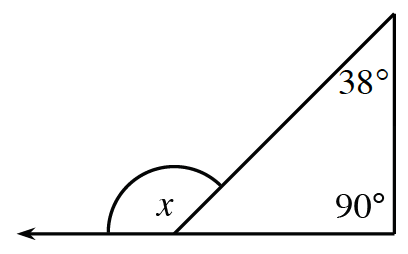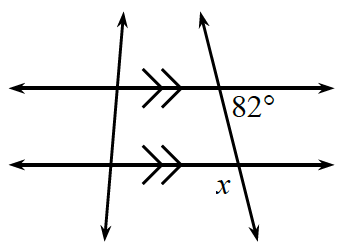### Home > CC3 > Chapter Ch10 > Lesson 10.1.4 > Problem10-61

10-61.

Calculate the value of $x$.

1.Angle $x$ is an exterior angle.

An exterior angle is equal to the sum of the two remote interior angles.

1.Vertical angles are always congruent. The angle that is vertical to $x$ is also supplementary to the given angle.

$x = 98º$